Applied and Computational Mathematics
Volume 6, Issue 4-1, July 2017, Pages: 48-54

Comparison of Singular Perturbations Approximation Method and Meta-Heuristic-Based Techniques for Order Reduction of Linear Discrete Systems

Anouar Bouazza

Department of Electrical Engineering, National Engineering School of Monastir, Sousse, Tunisia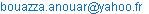Anouar Bouazza. Comparison of Singular Perturbations Approximation Method and Meta-Heuristic-Based Techniques for Order Reduction of Linear Discrete Systems. Applied and Computational Mathematics. Special Issue: Some Novel Algorithms for Global Optimization and Relevant Subjects. Vol. 6, No. 4-1, 2017, pp. 48-54. doi: 10.11648/j.acm.s.2017060401.14

Received: August 26, 2016; Accepted: September 12, 2016; Published: December 8, 2016

Abstract: This paper presents a survey of Singular Perturbations Approximation (SPA) method and meta-heuristic techniques for order reduction of linear systems in discrete case. A comparison of intelligent techniques to determine the reduced order model of higher order linear systems is presented. Two approaches are considered: Particle Swarm Optimization (PSO) and Shuffled Frog Leaping Algorithm (SFLA). These methods are employed to reduce the higher order model and based on the minimization of the Mean Square Error (MSE) between the transient responses of original higher order model and the reduced order model pertaining to a unit step input. Each method is illustrated through numerical examples.

Keywords: Order Reduction Techniques, Singular Perturbations Approximations Method, Meta-Heuristics Methods, Particle Swarm Optimization, Shuffled Frog Leaping Algorithm

Contents

1. Introduction

Reduction of high order systems (HOS) to lower order models has been an important subject area in control engineering for many years. The exact analysis of high order systems is both tedious and costly. The problem of reducing a HOS to its reduced order model (ROM) is considered important in analysis, synthesis and simulation of practical systems.

The model order reduction problem has been investigated in literature extensively, , , , , , , , , , , , , , several methods are available for large-scale system modeling . Most of the conventional methods, developed so far, are mostly available in continuous domain . However, the high order systems can be reduced in continuous as well as in discrete domain.

In recent years, one of the most promising research fields has been "Evolutionary Techniques" , , an area utilizing analogies with nature or social systems. These methods are stochastic search techniques with biological foundation.

Recently, Particle Swarm Optimization (PSO) and Shuffled Frog Leaping Algorithm (SFLA) appeared as a promising algorithm for handling the optimization problems in discrete case.

PSO  is a population-based stochastic optimization technique, inspired by social behavior of bird flocking or fish schooling. PSO shares many similarities with the Genetic Algorithms (GAs) , such as initialization of population of random solutions and search for the optimal by updating generations.

However, unlike GAs, PSO has no evolution operators, such as crossover and mutation. One of the most promising advantages of PSO over the GAs is its algorithmic simplicity when it uses a few parameters and is easy to implement.

Instead of using genes in GAs, SFLA  uses memes to improve spreading and convergence ratio. SFLA, in essence, combines the benefit of the local search tool of PSO and the idea of mixing information from parallel local searches, to move toward a global solution which is called a Shuffled Complex Solution.

This paper presents the application and performance comparison of SPA method, PSO and SFLA optimization techniques, for order reduction of linear systems in discrete case.

2. Order Reduction Techniques

Given a high order discrete time stable system of nth-order,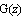, that is described by the z-transfer function: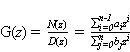(1)

where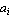and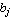are scalar constants.

The purpose is to find a reduced rth-order model,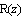, that has a transfer function (r < n):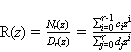(2)

where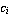and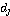are scalar constants.

The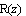approximates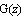in some sense and retains the important characteristics of.

2.1. Singular Perturbation Approximation Method

In mathematics, more precisely in perturbation theory, a singular perturbation problem is a problem containing a small parameter that cannot be approximated by setting the parameter value to zero. This is in contrast to regular perturbation problems, for which an approximation can be obtained by simply setting the small parameter to zero.

The perturbation methods  are commonly used by mathematicians to approach the equations of many systems. They provide solutions approached by switching to the limit close to the real system. The approach by the method of multi-time scale systems, or singular perturbation ,  enables the supply of simplified models by decoupling slow and fast dynamics of the global system and guarantees the fact that each model takes into account the dynamics of the other.

The discrete systems with the property of two-time scale ,  and can be written under the following form: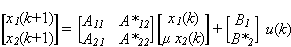(3)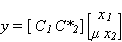(4)

with: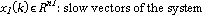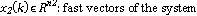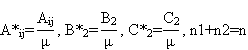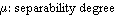.

The main difficulty of singular perturbation method is to show the small μ parameter of way to describe the system in the standard form. The separation of states, into those are slow and those are fast, is generally non trivial. A permutation and/or scaling of states are required to obtain separable model.

Some analytical methods of dynamics identification and iterative techniques allow, in most cases, to get around this difficulty.

The verification of two-time scale conditions (5) requires a packaging of the characteristic matrix’s system. The arrangement often requires changes such as permutation and calibration.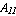invertible,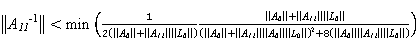(5)

with: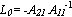The "arrow form characteristic" matrix  is proving very interesting as well for analysis and synthesis of systems that for the order reduction of the process.

Consider the following n-th order discrete time system: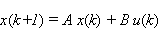(6)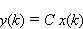(7)

where k is the time index,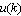is the input, x Rn is the state vector, u Rp and y Rm are the input and output vectors, respectively, and A Rn×n, B Rn×p, C Rm×n are matrices of appropriate dimensions with n, p and m being the system order, number of inputs and number of outputs respectively.

The corresponding desired reduced r-th order model is defined as follows: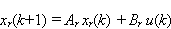(8)(9)

where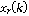is the r-state vector,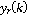is the reduced order model output of the system at the kth sampling instant and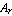,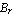and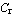are matrices with appropriate dimensions.

Using SPA method the reduced model is obtained as follows :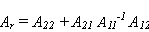(10)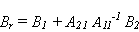(11)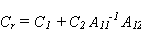(12)

To test the performance of the previous method, we propose a comparison with meta-heuristic-based techniques for order reduction.

2.2. Meta-Heuristic-Based Techniques for Order Reduction

In conventional mathematical optimization techniques, problem formulation must satisfy mathematical restrictions with advanced computer algorithm requirement, and may suffer from numerical problems. Further, in a complex system consisting of number of controllers, the optimization of several controller parameters using the conventional optimization is very complicated process and sometimes gets struck at local minima resulting in sub-optimal controller parameters.

Recently, meta-heuristics-based techniques for order reduction have appeared ,  and are now ahead of the conventional methods, they are generally based on PSO and SFLA. The process steps of the meta-heuristic-based techniques procedure, for model order reduction, are illustrated by the block diagram shown in Figure 1.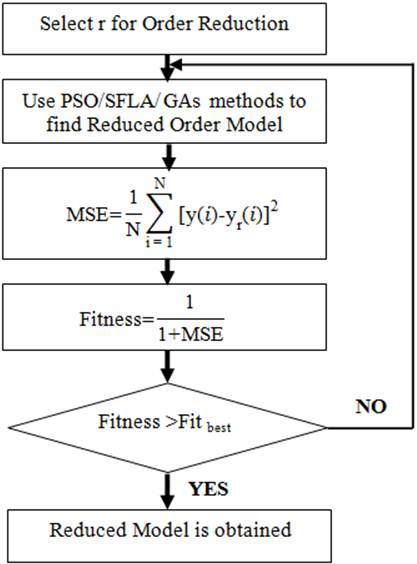Figure 1. Meta-heuristic methods for model order reduction procedure.

2.3. Overview of Particle Swarm Optimization Method

In recent years, one of the most promising research field has been "Heuristics from Nature", an area utilizing analogies with nature or social systems.

The Particle Swarm Optimization , ,  is an evolutionary computational model that optimizes a problem by improving a candidate solution. It is a population-based search algorithm where each individual is referred to as particle and represents a candidate solution. These particles are attracted towards the best solution found by particle’s neighborhood and by the particle.

Each particle has a position and velocity vector representing the potential solution to the problem.

The PSO use the following equations to update its velocity and position :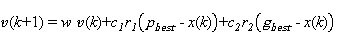(13)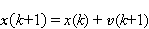(14)

where x and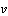represent the velocity and the position of the particle, respectively,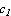and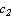are positive constants referred to as acceleration constants;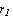and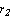are random numbers between 0 and 1;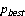refers to the best position found by the particle and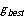refers to the global best position and finally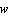is the inertia weight.

The computational flow chart of PSO algorithm, employed in the present study for the model reduction, is shown in Figure 2.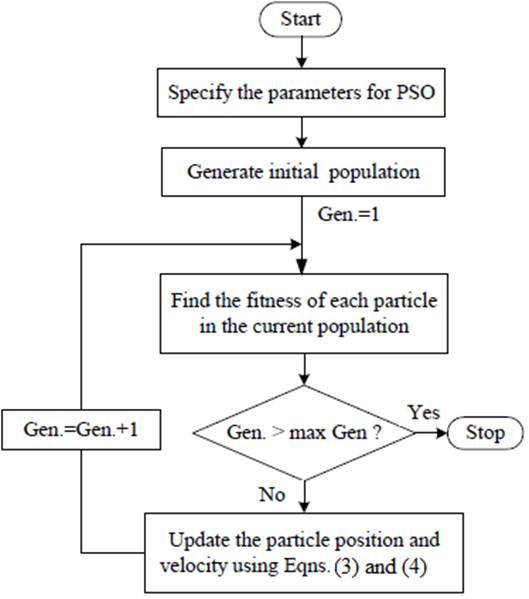Figure 2. Flowchart of PSO for order reduction.

2.4. Overview Shuffled Frog Leaping Algorithm

The Shuffled Frog Leaping Algorithm method is a member of wide category of swarm intelligence methods for solving the optimization problems presented for the first time by Eusuff and Lansey in 2003 . SFLA is combination of "meme-based genetic algorithm" or "Memetic Algorithm" and Particle Swarm Optimization. This algorithm has been inspired from memetic evolution of a group of frogs when seeking for food. In this method, a solution to a given problem is presented in the form of a string, called "frog".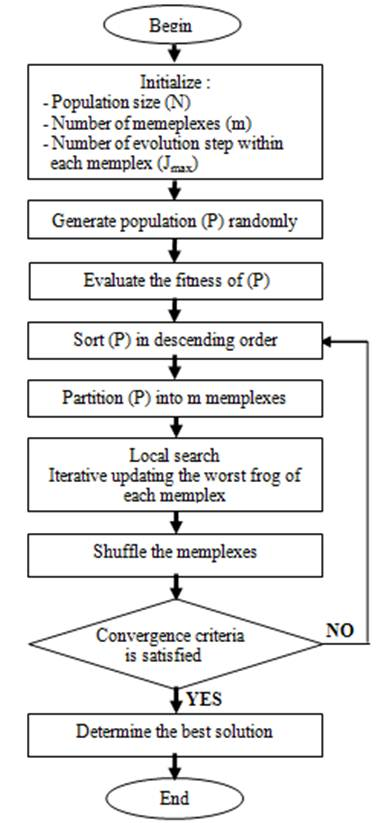Figure 3. Flowchart of SFLA.

The initial population of frogs is partitioned into groups or subsets called "memeplexes" and the number of frogs in each subset is equal. SFLA is based on two search techniques: local search and global information exchange techniques . Based on local search, the frogs in each subset improve their positions to have more foods (to reach the best solution). In second technique, obtained information between subsets is compared to each other (after each local search in subsets). The algorithm is iterative according to the flowchart illustrated in Figure 3.

The initial population is generated randomly. Then, the frogs are sorted in a descending order according to their fitness. Afterward, the entire population is partitioned into m mempelexes and the number of frogs in each memeplex is equal. In this process, the first frog goes to the first memeplex, the second frog goes to the second memeplex, the frog m goes to the m-th memeplex, and frog m+1 goes to the first, etc. The next step is based on local search. Within each memeplex, the frogs with the worst, the best and global best fitness are identified as Xw, Xb and Xg respectively. Then the position of the worst frog is adjusted as follows: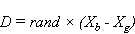(15)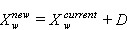(16)

where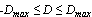, rand is a random number in the range of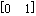and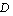is the step size vector and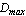is the maximum allowed change in a frog’s position.

If the new frog position does not improve, then equations (15) and (16) are repeated with respect to the global best frog (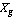replaces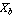). If no improvement becomes impossible in this latter case, then a random frog is generated to replace the old frog position. The shuffling process continues until convergence criteria are satisfied.

3. Experimental Results

3.1. Comparison of Methods

PSO and SFLA techniques have attracted considerable attention among various optimization techniques. Since the three approaches are supposed to find a solution to a given objective function but employ different strategies and computational effort, it is appropriate to compare their performance.

The estimation process is performed-based on the calculation of the fitness function defined as: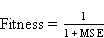(17)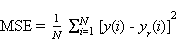(18)

and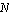is the number of elements in the output vectors,and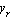are the original and reduced order models' responses respectively.

The above procedures for SPA method, PSO and SFLA in the present work is implemented in MATLAB. A number of examples were solved using the above given procedures and the results were satisfactory.

Altogether two examples are given in this section: 8th order and 4th order complex systems in discrete case. The results in the form of step response are shown in figures 4 and 6 and the comparison of "normalized fitness to 1" between the unit step responses of original and reduced systems obtained using proposed methods have been given in tables 3 and 4 for all the examples.

3.2. Example 1

To illustrate the proposed techniques and evaluate the performances of all approaches in this paper, consider the following 8th order complex discrete system given by :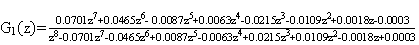(19)

From the transfer function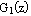we deduced the state-space model of the 8th order discrete system described by equations (6) and (7):(20)

It is clear that the matrix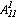is invertible and the two-time scale conditions (5) are verified.

The initial parameters for PSO and SFLA methods are given in tables 1 and 2.

Table 1. Initial parameters of PSO.

 Parameters Values Population size 100 c1 1.5 c2 1.5 wmax 0.9 wmin 0.4

Table 2. Initial parameters of SFLA.

 Parameters Values Number of memeplexes 10 Number of frog in each memplex 10 Number of iteration in each memplex 10

Using SPA method, PSO and SFLA methods, a 2nd order reduced model is obtained, as seen in Table 3 along with other reduced model of recently published work .

In addition to that, simulating the initial and reduced models is performed, the results, in the form of step responses, are seen in Figure 4 and the comparison of fitness "normalized to 1", between the unit step responses of original and reduced systems obtained using proposed meta-heuristic methods, have been given in Table 3.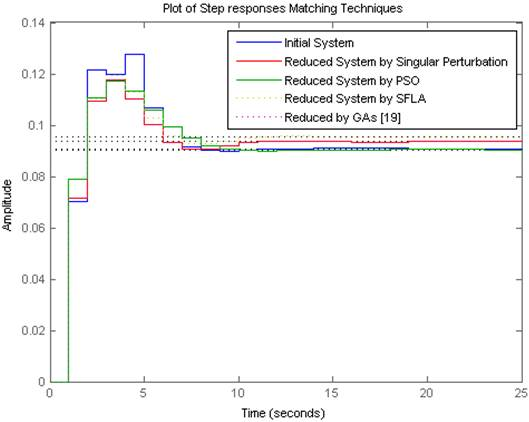Figure 4. Step responses comparison between original and reduced order models of example 1.

From the Figure 4 it is observed that the step responses of evolutionary reduced order models using PSO and SFLA methods are closely matching with the step response of higher order original system.

The figures 5 and 7 give an indication of fitness progress and speed convergence of objective function with the number of generations matching PSO and SFLA approaches.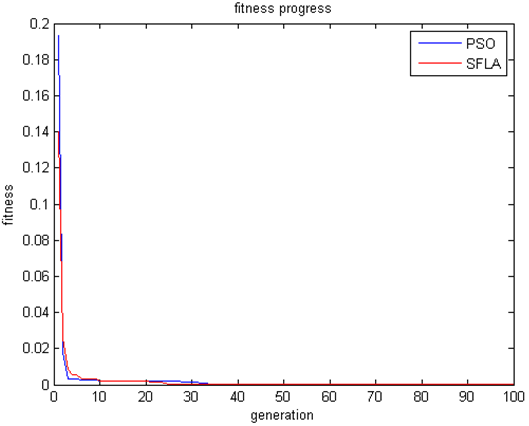Figure 5. Convergence of fitness function of example 1.

The superiority of the meta-heuristic PSO and SFLA approaches can be clearly seen where the best and average fitness "normalized to 1" are more important, close to 1, compared to SPA method seen in Table 3.

Table 3. Comparison of reduced order models.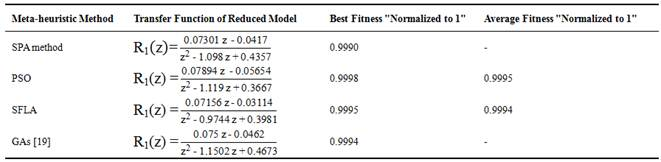3.3. Example 2

Consider, an another example, a 4th order complex system given by :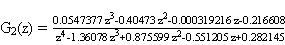(21)

From the transfer function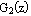we deduced the state-space model of the 4th order discrete system described by equations (6) and (7):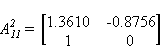(22)

It is clear that the matrix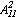is invertible and the two-time scale conditions (5) are verified.

The initial parameters for PSO and SFLA methods are given in tables 1 and 2.

A 2nd order reduced model is obtained, as seen in Table 4 along with other reduced model of recently published work .

In addition to that, simulating the initial and reduced models is performed, the results, in the form of step responses, are seen in Figure 6 and the comparison of fitness "normalized to 1", between the unit step responses of original and reduced systems obtained using proposed meta-heuristic methods, have been given in Table 4.

Table 4. Comparison of reduced order models.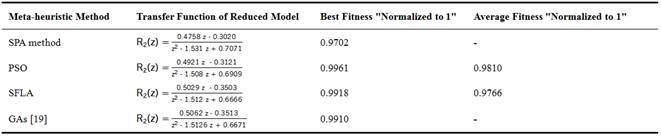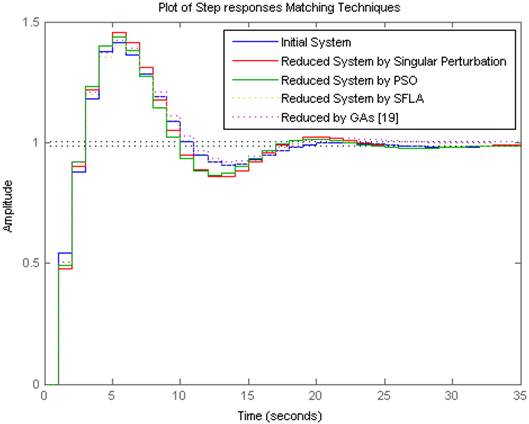Figure 6. Step responses comparison between original and reduced order models of example 2.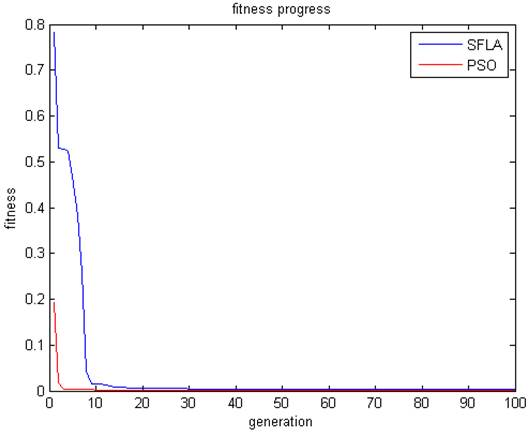Figure 7. Convergence of fitness function of example 2.

From Figure 6, it can be seen that the step responses of the proposed reduced order models are exactly matching with the higher order original system. The responses of evolutionary reduced model by PSO and SFLA are very close to that of original model.

The superiority of the meta-heuristic PSO and SFLA approaches can be clearly seen in Table 4 where the performance index, the best and average fitness "normalized to 1", of PSO and SFLA methods is compared with SPA method.

4. Conclusion

In this paper, three meta-heuristics approaches for reducing a high order large scale linear system into a lower order system have been proposed. Particle Swarm Optimization and Shuffled Frog Leaping Algorithm-based evolutionary optimization techniques are employed for the order reduction to attain reduced order models. The obtained results are compared with a recently published and existing well known method of model order reduction, the Genetic Algorithms, and with Singular Perturbations Approximation method to show their superiority.

It is clear, from results presented, that both PSO and SFLA methods give minimum Mean Square Error, i.e. fitness "normalized to 1", compared to GAs order reduction technique  and SPA method. Also, a comparison of the proposed methods has been presented. It is observed that both the proposed methods preserve steady state value and stability in the reduced models and the error between the initial or final values of the responses of original and reduced order models is very less. However, PSO method seems to achieve better results in view of its simplicity, easy implementation and better response.

Improvement of the quality of reduced order model obtained using PSO and SFLA methods in case of linear discrete systems using the proposed method was expected, but in order to use the approach further for more varieties of systems as well as for application of reduced order models in different areas, specific systems have to be modeled and the same need to be studied using the proposed method.

The methods are applied for real, complex and discrete systems and the work is in progress to make them generalized for continuous systems.

References

1. A. Bouazza, A. Sakly, and M. Benrejeb, "Order Reduction of Complex Systems described by TSK Fuzzy Models based on Singular Perturbations Method," International Journal of Systems Science, vol. 44. no. 3, pp. 442–449, March 2013.
2. A. Bouazza, A. Sakly, and M. Benrejeb, "On order reduction of discrete complex systems described by TSK fuzzy models," the9th International conference on Sciences and Techniques of Automatic control & computer engineering, Sousse, Tunisia,2008.
3. A. Bouazza, A. Sakly, and F. M'sahli, "Comparison of Different Meta-heuristic-based Techniques for Order Reduction of Linear Systems," the13th International conference on Sciences and Techniques of Automatic control & computer engineering, Monastir, Tunisia,2012.
4. V. Singh, D. Chandra, and H. KarI, "Improved Routh-Pade Approximants: A Computer-Aided Approach," IEEE Trans. Auto. Control, vol. 49. no.2, pp. 292-296, 2004.
5. M. Frangos and I. M. Jaimoukha, "Rational interpolation: Modified rational Arnoldi algorithm and Arnoldi-like equations," 46th IEEE Conference on Decision and Control, New Orleans LA, USA, 2007.
6. S. Devi and R. Prasad, "Reduction of Discrete time systems by Routh approximation," National System Conference, pp. 30-33, IIT Kharagpur, 2003.
7. L. Coluccio, A. Eisinberg, and G. Fedele, "A Prony-like polynomial-based approach to model order reduction, 15th Mediterranean conference on control & automation, 2007.
8. J. Lubuma and K. Patidar, "Towards the implementation of the singular function method for singular perturbation problems,"Applied mathematics and computation, 2009.
9. C. B. Vishwakarma and R. Prasad, "Clustering Method for Reducing Order of Linear System using Pade Approximation," IETE Journal of Research, vol. 54, Issue 5, pp. 326-330, 2008.
10. C. S. Hsieh and C. Hwang, "Model reduction of linear discrete-time systems using bilinear Schwarz approximation".J. Systems Sci, no. 1, pp. 33-49, 1990.
11. G. Parmar, S. Mukherjee, and R. Prasad, "System reduction using factor division algorithm and eigen spectrum analysis," Applied Mathematical Modelling, pp. 2542-2552, 2007.
12. G. Saraswathi, G.A. Gopala Rao, and Amarnath, "A Mixed method for order reduction of interval systems having complex eigenvalue," International Journal of Engineering and Technology, vol. 2, no. 4, pp. 201-206, 2008.
13. R. Prasad, A. K. Mittal, and S. P. Sharma, "A mixed method for the reduction of multi-variable systems," Journal of the Institution of Engineers, vol. 85, pp. 177-18, 2005.
14. O. M. K. Alsmadi and M. O. Abdalla, "Order Model Reduction for Two-Time-Scale Systems Based on Neural Network Estimation," 15th Mediterranean conference on Control & automation, 2007.
15. R. Sampaio and C. Soize, "Remarks on the efficiency of POD and KL methods for model reduction in nonlinear dynamics of continuous systems," International Journal for Numerical Methods in Engineering, 2006.
16. J. S. Yadav, N. P. Patidar, J. Singhai, S. Panda, and C. Ardil, "A Combined Conventional and Differential Evolution Method for Model Order Reduction," International Journal of Computational Intelligence, vol. 5, no. 2, pp. 111-118, 2009.
17. F. H. Bellamine and A. Elkamel, "Model order reduction using neural network principal component analysis and generalized dimensional analysis," International Journal for Computer-Aided Engineering and Software, 2008.
18. J. Kennedy and R. C. Eberhart, "Particle swarm optimization," IEEE International Conference on Neural Networks, Piscataway, pp. 1942-1948, 1995.
19. Satakshi, S. Mukherjee, and R.C. Mittal, "Order reduction of linear discrete systems using a genetic algorithm," Applied Mathematical modeling, Vol. 28, Issue 11, pp. 983-1005, November 2004.
20. N. H. Chahkandi, R. Jahani, and G.R. Sarlak, G, "Applying Shuffled Frog Leaping Algorithm for Economic Load Dispatch of Power System," American Journal of Scientific Research, Issue 20 pp. 82-89, 2011.
21. D. Soudani, "Sur la détermination explicite de solutions à des problèmes d’analyse et de synthèse de systèmes singulièrement perturbés, Application à un générateur de vapeur d’un navire," Thèse de doctorat, ENIT Tunisia, 1997.
22. M. N. Abdelkrim, "Sur la modélisation et la synthèse des systèmes singulièrement perturbés. Application aux processus dynamiques," Thèse de doctorat, ENIT, Tunisia, 1985.
23. G. Dauphin-Tanguy, P. Borne, and A. Fossard, "Analyse et synthèse des systèmes à plusieurs échelles de temps," Journal d’étude, pp. 169-196, 1985.
24. Z. S.Abo-Hammour, O.M. K. Alsmadi, and A. M. AlSmadi, "Frequency-based model order reduction via genetic algorithm approach,"Systems, Signal Processing and their Applications (WOSSPA), 7th International Workshop on, IEEE Xplore, pp. 91-94, 2011.
25. S. Panda, S.K. Tomar, R. Prasad, and C. Ardil, " Model Reduction of Linear Systems by Conventional and Evolutionary Techniques," International Journal of Computational and Mathematical Sciences, vol. 3, no. 1, pp. 28-34, 2009.
26. S. Panda, J.S. Yadav, N.P. Patidar, and C. Ardil, "Evolutionary Techniques for Model Order Reduction of Large Scale Linear Systems," International Journal of Applied Science, Engineering and Technology, vol. 5, no. 1, pp. 22-28, 2009.
27. S. Panda, N. P. Padhy, and R. N. Patel, "Power System Stability Improvement by PSO Optimized SSSC-based Damping Controller," Electric Power Components & Systems, Taylor and Francis, vol. 36, no. 5, pp. 468-490, 2008.
28. S. Panda, N. P. Padhy, and R. N. Patel, "Robust Coordinated Design of PSS and TCSC using PSO Technique for Power System Stability Enhancement," Journal of Electrical Systems, vol. 3, no. 2, pp. 109-123, 2007.
29. G. Pamar and S. Mukherjee, "Reduced order modeling of linear dynamic systems using Particle Swarm optimized eigen spectrum analysis," International journal of Computational and Mathematical Sciences, pp. 45-52, 2007.
30. M. Eusuff and K.E. Lansey, K. E, "Optimization of Water Distribution Network Design Using the Shuffled Frog Leaping Algorithm," J Water Resour Plan Manage, no. 3, pp. 10-25, 2003.
31. M. Tavakolan, A. Baabak, and N. Chiara, "Applying the Shuffled Frog-Leaping Algorithm to improve scheduling of construction projects with activity splitting allowed," Management and Innovation for a Sustainable Built Environment, pp. 20-23, 2011.

Biography

 Anouar Bouazza was born in Sousse-Tunisia in 1982. He got his National Diploma of Engineer in 2006 and Master Diploma in 2008 from the National Engineering School of Monastir (ENIM). He is currently preparing for his PhD "On Order Reduction of Complex Systems using conventional approaches and intelligent methods" in Electrical Engineering at ENIM.He is presently Teaching Assistant in Electrical Engineering and Computer Science at the Private Institute of Higher Studies (IHE Group) Sousse - Tunisia.
 Contents 1. 2. 2.1. 2.2. 2.3. 2.4. 3. 3.1. 3.2. 3.3. 4.
Article ToolsAbstractPDF(1087K)